Courses

# Technical Test SSC JE: Electrical Engineering (EE)- 1

## 100 Questions MCQ Test Electrical Engineering SSC JE (Technical) | Technical Test SSC JE: Electrical Engineering (EE)- 1

Description
This mock test of Technical Test SSC JE: Electrical Engineering (EE)- 1 for Electrical Engineering (EE) helps you for every Electrical Engineering (EE) entrance exam. This contains 100 Multiple Choice Questions for Electrical Engineering (EE) Technical Test SSC JE: Electrical Engineering (EE)- 1 (mcq) to study with solutions a complete question bank. The solved questions answers in this Technical Test SSC JE: Electrical Engineering (EE)- 1 quiz give you a good mix of easy questions and tough questions. Electrical Engineering (EE) students definitely take this Technical Test SSC JE: Electrical Engineering (EE)- 1 exercise for a better result in the exam. You can find other Technical Test SSC JE: Electrical Engineering (EE)- 1 extra questions, long questions & short questions for Electrical Engineering (EE) on EduRev as well by searching above.
QUESTION: 1

### Kirchhoff's second law is based on the law of conservation of

Solution:

This law is based on the conservation of energy whereby voltage is defined as the energy per unit charge. The total amount of energy gained per unit charge must be equal to the amount of energy lost per unit charge, as energy and charge are both conserved.

QUESTION: 2

Solution:
QUESTION: 3

### For the circuit shown the reading in the ammeter A will be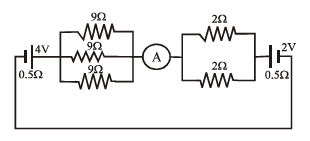Solution: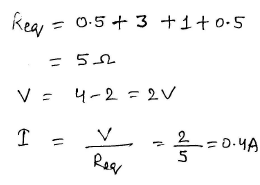QUESTION: 4

When checked with an ohm meter an open resistor reads

Solution:

If you don't connect the probes to anything, you have an open circuit, there is no path from one to the other (except through the air), and the meter will read infinity ohms.

QUESTION: 5

A light dependent resistor is basically a

Solution:

A light dependent resistor abbreviated as LDR is an electronic device which senses light. It is basically a variable resistor whose value varies with the intensity of incident light. It consists of a pair of metal contacts between which a curved track of cadmium sulphide is placed

QUESTION: 6

Voltage dependent resistors are usually made from

Solution:
QUESTION: 7

Resistors across A & B in the circuit shown below is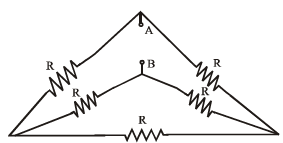Solution:

On rearranging, we get a balanced wheatstone bridge. hence the effective resistance is R

QUESTION: 8

Voltage dependent resistors are used

Solution: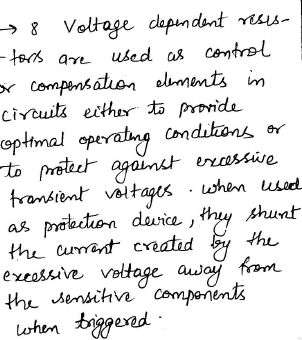QUESTION: 9

The power factor of incandescent bulb is

Solution:

In incandescent bulb there is filament, which is purely restive in nature. and we know that purely resistive element has unity power factor.

QUESTION: 10

The equivalent inductance at seen at terminal AB is_____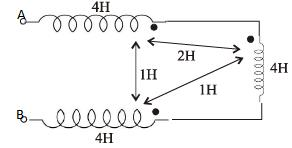Solution:
QUESTION: 11

If V2 = 1 – e–2t, the value of V1 is given bySolution: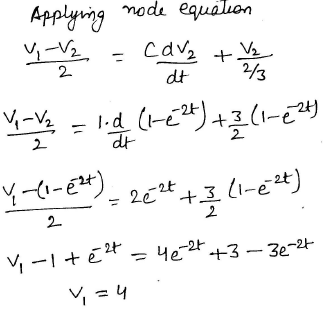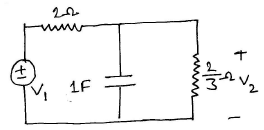QUESTION: 12

For a parallel RLC circuit to be overdamped

Solution: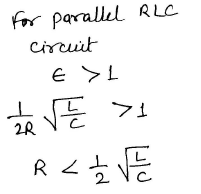QUESTION: 13

The thevenin resistance across the diode in the circuit is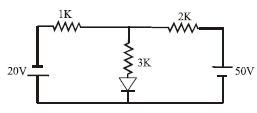Solution: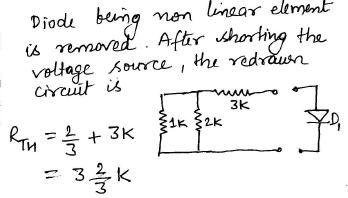QUESTION: 14

Find input resistance of the circuit shown is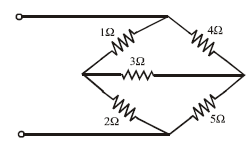Solution: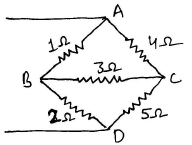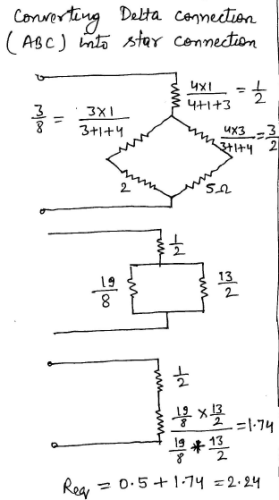QUESTION: 15

A 3H – Inductor has 2000 turns. How many turns must be added to increase the inductance to 5H

Solution: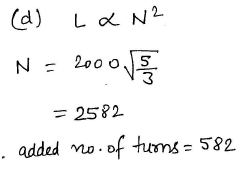QUESTION: 16

For a series RLC circuit the power factor at the lowest half power frequency is

Solution:
QUESTION: 17

A series RLC circuit resonance at 1MHz at frequency of 1.1 MHz the circuit impedance is

Solution:
QUESTION: 18

If each branch of a delta circuit has impedance √3z , then each branch of equivalent Y–circuit
has impedance

Solution: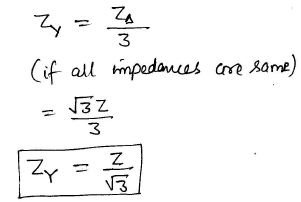QUESTION: 19

A delta load is connected to a balanced 400 V, 3-f supply as shown in figure. The total power dissipated in the network is equal to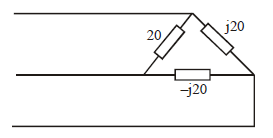Solution: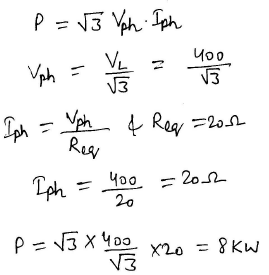QUESTION: 20

When Q-factor of the circuit is high, then

Solution:
QUESTION: 21

The dynamic resistance of a parallel resonance circuit is given by

Solution:
QUESTION: 22

A parallel resonant circuit can be employed

Solution:
QUESTION: 23

A 3-f star connected symmetrical load consumes p watts of power from a balancedsupply. If the same load is connected in delta to the same supply, the power consumption will be

Solution:
QUESTION: 24

In two wattmeters method of power measurement, one of the wattmeters will show negative reading when the load power factor angle is strictly

Solution:

The reading of a wattmeter is proportional to (current through the current coil)x(voltage across the pressure coil)xCos(angle between this current and this voltage).

So if this angle becomes more than 90 degrees the the reading of the wattmeter will be negative.This could happen in a 3-ph unbalanced circuit.

For a 3-ph balanced load the two wattmeter readings will be,say Vab*Ia*cos (30+phi) and Vcb*Ic* cos(30-phi). So if phi >60 degrees (pf less than 0.5) a negative reading is obtained.

QUESTION: 25

The external characteristics of a shunt generator can be obtained directly from its ____characteristics

Solution:
QUESTION: 26

For the voltage build up of a self excited d.c. generator, which of the following is not anessential condition?

Solution:
QUESTION: 27

Which of the following d.c. generator cannot build up on open-circuit?

Solution:
QUESTION: 28

Which of the following methods of speed control of D.C. machine will offer minimum efficiency?

Solution:
QUESTION: 29

Which winding of the transformer has less crosssectional area?

Solution:

High voltage winding because coil thickness is low comparing to others

QUESTION: 30

A dc series motor of resistance 1Ω across terminal runs at 1000 rpm at 250V taking acurrent of 20A when an additional resistance 6W is inserted in series and taking the samecurrent, the new speed will be

Solution: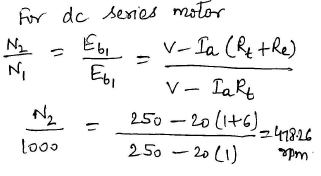QUESTION: 31

In dc motors, the condition for maximum power is

Solution:

The condition for maximum power in case of D.C. motor is

Supply voltage =I × back e.m.f. D.

QUESTION: 32

The speed of a series wound dc motor

Solution:
QUESTION: 33

In dc series motor 1 shaft torque is less than the armature torque due to

Solution:
QUESTION: 34

A dc shunt motor has rated rpm of 480 contain industrial application require this motor to runat 540 rpm for sometime which speed control will be desirable

Solution:
QUESTION: 35

The leakage flux in a transformer depends upon

Solution:
QUESTION: 36

In a transformer if peak voltage is fed to primary

Solution:
QUESTION: 37

The phase difference between the primary & secondary voltage of a transformer is

Solution:

There is 90 degree phase shift between voltage and magnetic field. So primary voltage causes 90 degree phase shifted magnetic field, which induces again 90 degree phase shifted secondary voltage. So it adds up to 180 degree. If you change winding direction, secondary voltage will now cancel that phase shift and you will get 0 degree phase shift.

QUESTION: 38

All of the following methods/techniques can be used for the measurement of high ac voltages EXCEPT

Solution:
QUESTION: 39

Consider the circuit shown in the given figure for maximum power transfer to the load, the primary to secondary turn ratio must be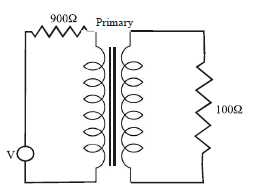Solution:
QUESTION: 40

Maximum electric power output of a synchronous generator is

Solution:

The power developed in cylindrical synchronous machine is Maximum power developed Pmax = EV/Xs Where E = Excitation voltage V = Terminal voltage Xs = Synchronous reactance δ = load angle Therefore, maximum power developed in cylindrical synchronous machine is at a load angle of 90°.

QUESTION: 41

Two alternators are running in parallel. If the field of one of the alternator is adjusted, it will

Solution:

If the field excitation (voltage adjustment control)of one of a pair of paralleled alternators is turned down, two things will happen: first, the generator in question will develop a less lagging power factor, while the other generator will develop a more lagging power factor, with the overall power factor of the plant and load remaining the same; second, bus voltage, and by extension grid voltage, will drop slightly, unless the other generator’s voltage adjustment (field excitation) is increased proportionally, which will also result in the second generator develop an even more lagging power factor as the first generator’s power factor becomes less lagging.

QUESTION: 42

An unexcited single phase synchronous motor is

Solution:
QUESTION: 43

A synchronous motor is switched on to supply with its field winding shorted on themselves it will

Solution:
QUESTION: 44

The power factor of pure resistive circuit is

Solution:
QUESTION: 45

Under which method of starting an induction motor is expected to take largest starting current?

Solution:

In Direct on line starting, an induction motor is expected to take largest starting current.

In Direct on line starting (DOL) , the contactor will be controlled by separate start and stop buttons, and an auxiliary contact on the contactor is used, across the start button, as a hold in contact. i.e. the contactor is electrically latched closed while the motor is operating.

QUESTION: 46

A dc motors develops a torque of 200 N-m at 25 rps. At 20 rps, it will develop a torque of____ N-m

Solution: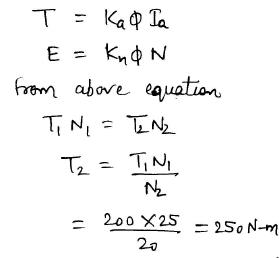QUESTION: 47

An eight pole wound rotor induction motor operating on 60 Hz supply is driven at 1800rpm, by a prime mover in the opposite direction of revolving magnetic field. The frequency ofrotor current is

Solution: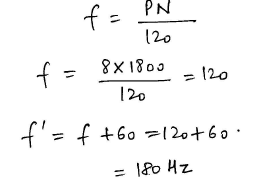QUESTION: 48

A 6-pole, 50 Hz, 3-f induction motor is running at 950 rpm and has rotor Cu loss of 5kw. Its rotor input is ____ kw

Solution: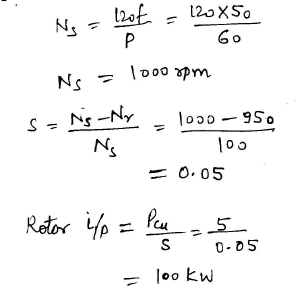QUESTION: 49

A 6 pole, 50 hz-, 3-f induction motor has a full load speed of 950 rpm. At half load, its speed would be ____ rpm

Solution: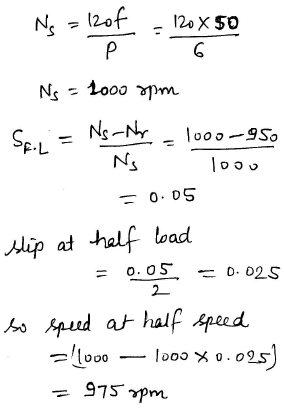QUESTION: 50

A 60 w lamp given a luminous flux of 1500 lumen. Its efficiency is

Solution:
QUESTION: 51

Which of the following is a cold cathode lamps?

Solution:
QUESTION: 52

Which of the following lamp has least capacity to sustain voltage fluctuations?

Solution:

Effect of Voltage variation in incandescent lamp

Within the normal range of supply voltage variations the light output will change by about 3.5% for a 1% change of voltage. The effect of voltage on life is much more pronounced; 5% over-voltage will roughly halve the lamp life, whereas 5% under-voltage will approximately double it.

QUESTION: 53

Unbalanced forces are maximum in case of

Solution:
QUESTION: 54

Which motor is used in tramways

Solution:

Mostly DC series motors are used in this system. For trolley buses and tramways, DC compound motors are used where regenerative braking is required. The various operating voltages of DC traction system include 600V, 750 V, 1500V and 3000V.

QUESTION: 55

In arc welding, the voltage on ac supply system is in the range

Solution:
QUESTION: 56

In gas welding the gases used are

Solution:

Gases used in welding and cutting processes include: shielding gases such as carbon dioxide, argon, helium, etc. fuel gases such as acetylene, propane, butane, etc. oxygen, used with fuel gases and also in small amounts in some shielding gas mixtures.

QUESTION: 57

For welding duty, the rectifier commonly used are

Solution:

The rectifier types are commonly called "Welding Rectifiers" and produce DC or, AC and DC welding current. They may utilize either single phase or three phase input power. They contain a transformer, but rectify the AC or DC by the use of selenium rectifiers, silicon diodes or silicon controlled rectifiers.

QUESTION: 58

In hygrometers the principle of measurement is

Solution:

Hygrometer uses for measuring the humidity present in the surrounding environment. The term humidity means the amount of water vapour present in the gas. The physical properties of the material changes by the effect of the humidity and this principle use in hygrometer for measurement.

QUESTION: 59

A signal of 10 mv at 75 MHz is to be measured which of the following instrument can be used?

Solution:

At high frequencies, cathode ray oscilloscope  is used

QUESTION: 60

Holes are filled on the opposite side of the disc of an induction type energy meter to

Solution:
QUESTION: 61

Galvanometer type recorders use

Solution:

In a galvanometer-type recorder, the pointer of the D'Arsonval movement is fitted with a pen-ink (stylus) mechanism. The pointer deflects when current flows through the moving coil.

QUESTION: 62

The curve representing Ohm’s law is

Solution:

From Ohm’s law
V/I = K = R
V = IR
= Linear

QUESTION: 63

A slide wire potentiometer is used to measure voltage across a dc circuit. The voltage is 1.2V as read by the potentiometer. Across the same points 20000 W/V voltmeter reads 0.6V on its 5V range. Input resistance between the two point is

Solution: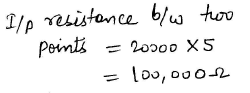QUESTION: 64

In a gravity controlled instrument, the deflection angle is proportional to

Solution:
QUESTION: 65

In a Q-meter measurement to determine the self capacitance of a coil, the first resonance occurred at F1 with C1 = 30 PF. The second resonance occurred at F2 = 2F1 with C2 = 60PF. The self capacitance of coil works out to be

Solution:
QUESTION: 66

The deflection of hot wire instrument depends on

Solution:

The deflection of hot wire instrument depends on RMS value of alternating current.

QUESTION: 67

Which one of the following types of instruments can be used to determine the rms value of a.c.voltage of high magnitude (10 KV) and of any wave shape?

Solution:

Electrostatic Instruments are used for voltage measurements only

QUESTION: 68

Eddy current damping is not used on type instruments because

Solution:
QUESTION: 69

Least expensive instrument for dc measurement is

Solution:
QUESTION: 70

A Meggar is basically a

Solution:
QUESTION: 71

The economic size of a conductor is determined by

Solution:

"The Kelvin's law states that the most economical size of a conductor is that for which annual interest and depreciation on the capital cost of the conductor is equal to the annual cost of energy loss."

QUESTION: 72

For a good voltage profile under load condition a long line needs

Solution:

Shunt capacitive compensation. This method is used to improve the power factor. Whenever an inductive load is connected to the transmission line, power factor lags because of lagging load current. To compensate, a shunt capacitor is connected which draws current leading the source voltage.

QUESTION: 73

An industrial consumer has a daily load pattern of 2000 kw, 0.8 p.f. for 12 Hrs and 1000 kw UPF 12 Hrs. The load factor is

Solution: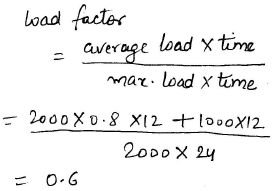QUESTION: 74

The positive sequence reactance will be equal to  the negative sequence reactance.. the equipment is

Solution:
QUESTION: 75

In overhead transmission lines the effect of capacitance can be neglected when the length of line is less than

Solution:
QUESTION: 76

Sparking occurs when a load is switched off because the circuit has

Solution:

Wires have inductance associated with it. When load is disconnected current through the wire changes instantaneously. Now inductors can not allow a sudden change in current through them. As a result high voltage grows at the open end of the wire causes breakdown in air. For this there is sparking. Spark plug operates under same principle. Although value of inductance is higher.

QUESTION: 77

Which relay is used for feeders?

Solution:
QUESTION: 78

Which of the following is a conducting medium for electric current?

Solution:
QUESTION: 79

Dielectric strength of SF6 is

Solution:

Explanation : The dielectric strength which is 30 % less than that of oil at atmospheric pressure increases rapidly with increase of pressure.

QUESTION: 80

Thermal relays are often used in

Solution:
QUESTION: 81

Differential protection principle is used in the protection of

Solution:

Differential relay is very commonly used relay for protecting transformers and generators from localised faults. Differential relays are very sensitive to the faults occurred within the zone of protection but they are least sensitive to the faults that occur outside the protected zone.

QUESTION: 82

A cable carrying alternating current has

Solution:
QUESTION: 83

Cables for 220 KV lines are invariably

Solution:
QUESTION: 84

In a cable, the voltage stress is maximum at

Solution:
QUESTION: 85

When diameter of the core and cable is doubled the value of capacitance

Solution:
QUESTION: 86

Which of the following method or technique can be used for the measurement of high dc voltages?

Solution:
QUESTION: 87

For the same voltage boost, the reactive power capacity is

Solution:
QUESTION: 88

With the help of a reactive compensator it is possible to have

Solution:
QUESTION: 89

A shunt reactor at 100 MVAr is operated at 98% of its rated voltage and at 96% of it srated frequency. The reactive power absorbed by the reactor is

Solution: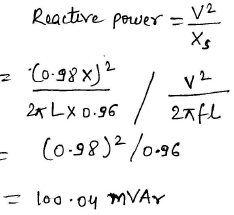QUESTION: 90

The junction capacitance of a p-n junction depends on

Solution:
QUESTION: 91

In p+n junction diode under reverse bias, the magnitude of electric field is maximum at

Solution:

Electric field is always maximum at the junction

QUESTION: 92

How can the channel width in a junction field effect transistor be controlled?

Solution:
QUESTION: 93

A Zener diode works on the principle of

Solution:

Due to zener effect in reverse bias under high electric field strength, electron quantum tunneling occurs. It’s a mechanical effect in which a tunneling current occurs through a barrier. They usually cannot move through that barrier.

QUESTION: 94

As compared to full wave rectifier using two diodes, the four diode bridge rectifier has the dominant advantage of

Solution:
QUESTION: 95

In a transistor, the forward bias across the base emitter junction is kept constant and the reverse bias across the collector base junction is increased. Neglecting, the leakage across the collector base junction & the depletion region generation current, the base current will

Solution:
QUESTION: 96

Thermal runaway in a transistor biased in active region is due to

Solution:
QUESTION: 97

The photo electric current in amperes per watt of incident light depend on

Solution:
QUESTION: 98

In PNP transistor, with normal bias, the emitter junction is

Solution:
QUESTION: 99

A transistor will operate in inverted region when

Solution:

Forward-active (or simply active)

The base–emitter junction is forward biased and the base–collector junction is reverse biased. Most bipolar transistors are designed to afford the greatest common-emitter current gain, βF, in forward-active mode. If this is the case, the collector–emitter current is approximately proportional to the base current, but many times larger, for small base current variations

Reverse-active (or inverse-active or inverted)

By reversing the biasing conditions of the forward-active region, a bipolar transistor goes into reverse-active mode. In this mode, the emitter and collector regions switch roles. Because most BJTs are designed to maximize current gain in forward-active mode, the βF in inverted mode is several times smaller (2–3 times for the ordinary germanium transistor). This transistor mode is seldom used, usually being considered only for failsafe conditions and some types of bipolar logic. The reverse bias breakdown voltage to the base may be an order of magnitude lower in this region.

QUESTION: 100

In a NPN transistor if the emitter junction is reverse biased and collector junction is reverse biased, the transistor will operate in

Solution: